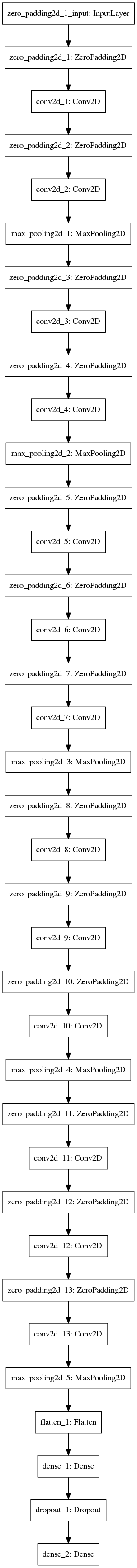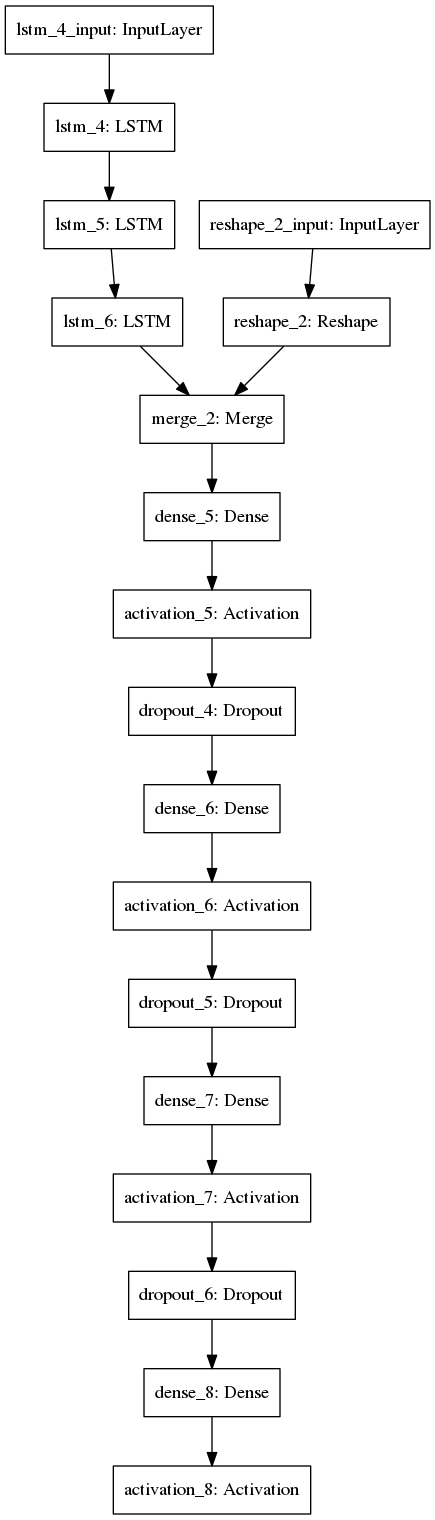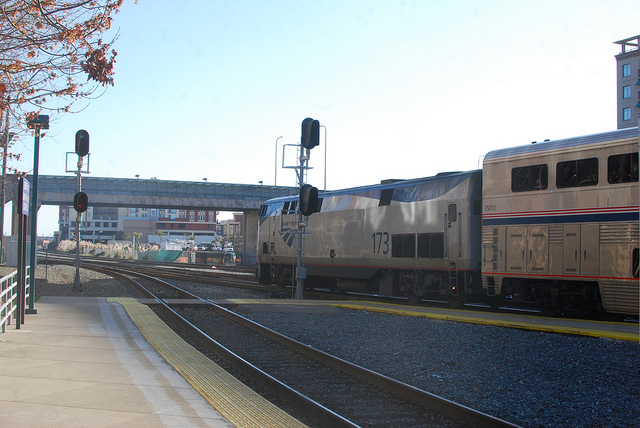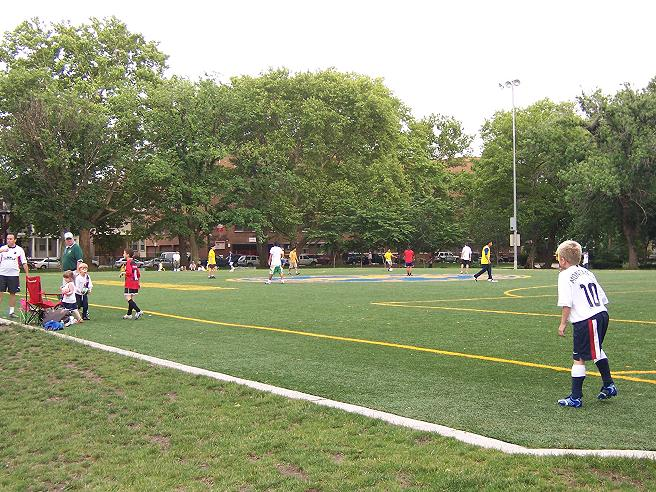# Visual Question Answering Demo in Python Notebook

This is an online demo with explanation and tutorial on Visual Question Answering. This is not a naive or hello-world model, this model returns close to state-of-the-art without using any attention models, memory networks (other than LSTM) and fine-tuning, which are essential recipe for current best results.

I have tried to explain different parts, and reasoning behind their choices. This is meant to be an interactive tutorial, feel free to change the model parameters and experiment. If you have latest graphics card execution time should be within a minute.

All the files required to run this ipython notebook can be obtained from

#### * https://github.com/iamaaditya/VQA_Demo * Jupyter Notebook on Github </p>

In :
%matplotlib inline
import os, argparse
import cv2, spacy, numpy as np
from keras.models import model_from_json
from keras.optimizers import SGD
from sklearn.externals import joblib


## Load the models and weights files¶

This does not load the models yet, but we are providing the files

In :
# File paths for the model, all of these except the CNN Weights are
VQA_model_file_name      = 'models/VQA/VQA_MODEL.json'
VQA_weights_file_name   = 'models/VQA/VQA_MODEL_WEIGHTS.hdf5'
label_encoder_file_name  = 'models/VQA/FULL_labelencoder_trainval.pkl'
CNN_weights_file_name   = 'models/CNN/vgg16_weights.h5'


## Model Idea¶

This uses a classical CNN-LSTM model like shown below, where Image features and language features are computed separately and combined together and a multi-layer perceptron is trained on the combined features.

Similar models have been presented at following links, this work takes ideas from them.Source

# Image features¶

## Pretrained VGG Net (VGG-16)¶

While VGG Net is not the best CNN model for image features, GoogLeNet (winner 2014) and ResNet (winner 2015) have superior classification scores, but VGG Net is very versatile, simple, relatively small and more importantly portable to use.

For reference here is the VGG 16 performance on ILSVRC-2012## Compile the model¶

In :
def get_image_model(CNN_weights_file_name):
''' Takes the CNN weights file, and returns the VGG model update
with the weights. Requires the file VGG.py inside models/CNN '''
from models.CNN.VGG import VGG_16
image_model = VGG_16(CNN_weights_file_name)

# this is standard VGG 16 without the last two layers
sgd = SGD(lr=0.1, decay=1e-6, momentum=0.9, nesterov=True)
# one may experiment with "adam" optimizer, but the loss function for
# this kind of task is pretty standard
image_model.compile(optimizer=sgd, loss='categorical_crossentropy')
return image_model


## Plot the Model¶

Keras has a function which allows you to visualize the model in block diagram. Let's do it !

In :
from keras.utils.visualize_util import plot
model_vgg = get_image_model(CNN_weights_file_name)
plot(model_vgg, to_file='model_vgg.png')## Extract Image features¶

Extracting image features involves, taking a raw image, and running it through the model, until we reach the last layer. In this case our model is not 100% same as VGG Net, because we are not going to use the last two layer of the VGG. It is because the last layer of VGG Net is a 1000 way softmax and the second last layer is the Dropout.

Thus we are extracting the 4096 Dimension image features from VGG-16

In :
def get_image_features(image_file_name, CNN_weights_file_name):
''' Runs the given image_file to VGG 16 model and returns the
weights (filters) as a 1, 4096 dimension vector '''
image_features = np.zeros((1, 4096))
# Magic_Number = 4096  > Comes from last layer of VGG Model

# Since VGG was trained as a image of 224x224, every new image
# is required to go through the same transformation
im = im.transpose((2,0,1)) # convert the image to RGBA

# this axis dimension is required because VGG was trained on a dimension
# of 1, 3, 224, 224 (first axis is for the batch size
# even though we are using only one image, we have to keep the dimensions consistent
im = np.expand_dims(im, axis=0)

image_features[0,:] = get_image_model(CNN_weights_file_name).predict(im)
return image_features


# Word Embeddings¶

The question has to be converted into some form of word embeddings. Most popular is Word2Vec whereas these days state of the art uses skip-thought vectors or positional encodings.

We will use Word2Vec from Stanford called Glove. Glove reduces a given token into a 300 dimensional representation.

In :
def get_question_features(question):
''' For a given question, a unicode string, returns the time series vector
with each word (token) transformed into a 300 dimension representation
calculated using Glove Vector '''
tokens = word_embeddings(question)
question_tensor = np.zeros((1, len(tokens), 300))
for j in xrange(len(tokens)):
question_tensor[0,j,:] = tokens[j].vector
return question_tensor


## Try the embeddings¶

Let's see the embeddings, and their usage with sample words like this -

1. Obama
2. Putin
3. Banana
4. Monkey
In :
word_embeddings = spacy.load('en', vectors='en_glove_cc_300_1m_vectors')

In :
obama = word_embeddings(u"obama")
putin = word_embeddings(u"putin")
banana = word_embeddings(u"banana")
monkey = word_embeddings(u"monkey")

In :
obama.similarity(putin)

Out:
0.43514112534149385
In :
obama.similarity(banana)

Out:
0.17831375020636123
In :
banana.similarity(monkey)

Out:
0.45207779162154438

As we can see, obama and putin are very similar in representation than obama and banana. This shows you there is some semantic knowledge of the tokens embedded in the 300 dimensional representation. We can do cool arithmetics with these word2vec like 'Queen' - 'King' + 'Boy' = 'Girl'. See this blog post for more details.

## VQA Model¶

VQA is a simple model which combines features from Image and Word Embeddings and runs a multiple layer perceptron.

In :
def get_VQA_model(VQA_model_file_name, VQA_weights_file_name):
''' Given the VQA model and its weights, compiles and returns the model '''

# thanks the keras function for loading a model from JSON, this becomes
# very easy to understand and work. Alternative would be to load model
# from binary like cPickle but then model would be obfuscated to users
vqa_model.compile(loss='categorical_crossentropy', optimizer='rmsprop')
return vqa_model

In :
from keras.utils.visualize_util import plot
model_vqa = get_VQA_model(VQA_model_file_name, VQA_weights_file_name)
plot(model_vqa, to_file='model_vqa.png')As it can be seen above the model also runs a 3 layered LSTM on the word embeddings. To get a naive result it is sufficient to feed the word embeddings directly to the merge layer, but as mentioned above the model gives close to the state-of-the-art results.

Also, four layers of fully connected layers might not be required to achieve a good enough results. But I settled on this model after some experimentation, and this model's results beat those obtained using only few layers.

Let's give a test image and a question

In :
image_file_name = 'test.jpg'
question = u"What vehicle is in the picture?"


# What vehicle is in the picture ? ¶In :
# get the image features
image_features = get_image_features(image_file_name, CNN_weights_file_name)

In :
# get the question features
question_features = get_question_features(question)

In :
y_output = model_vqa.predict([question_features, image_features])

# This task here is represented as a classification into a 1000 top answers
# this means some of the answers were not part of training and thus would
# not show up in the result.
# These 1000 answers are stored in the sklearn Encoder class
for label in reversed(np.argsort(y_output)[0,-5:]):
print str(round(y_output[0,label]*100,2)).zfill(5), "% ", labelencoder.inverse_transform(label)

78.32 %  train
01.11 %  truck
00.98 %  passenger
00.95 %  fire truck
00.68 %  bus


## Results¶

I am copying the output of the previous command, so that you can validate if your results are same as mine.

78.32 % train
01.11 % truck
00.98 % passenger
00.95 % fire truck
00.68 % bus

# Demo with image URL¶

Since cv2.imread cannot read an image from URL we will have to change our function get_image_features

In :
def get_image_features(image_file_name, CNN_weights_file_name):
''' Runs the given image_file to VGG 16 model and returns the
weights (filters) as a 1, 4096 dimension vector '''
image_features = np.zeros((1, 4096))

from skimage import io
# if you would rather not install skimage, then use cv2.VideoCapture which can read from URL
im = im.transpose((2,0,1)) # convert the image to RGBA

# this axis dimension is required because VGG was trained on a dimension
# of 1, 3, 224, 224 (first axis is for the batch size
# even though we are using only one image, we have to keep the dimensions consistent
im = np.expand_dims(im, axis=0)

image_features[0,:] = get_image_model(CNN_weights_file_name).predict(im)
return image_features

In :
image_file_name = "http://www.newarkhistory.com/indparksoccerkids.jpg"
# get the image features
image_features = get_image_features(image_file_name, CNN_weights_file_name)


Feel free to change that url to any valid image, it can be any image format. Also try to use websites which have higher bandwidth# What are they playing? ¶

In :
question = u"What are they playing?"

# get the question features
question_features = get_question_features(question)

In :
y_output = model_vqa.predict([question_features, image_features])

for label in reversed(np.argsort(y_output)[0,-5:]):
print str(round(y_output[0,label]*100,2)).zfill(5), "% ", labelencoder.inverse_transform(label)

40.52 %  tennis
28.45 %  soccer
17.88 %  baseball
11.67 %  frisbee
00.15 %  football


## Result¶

Copying the result to validate your output.

40.52 % tennis
28.45 % soccer
17.88 % baseball
11.67 % frisbee
00.15 % football

As you can see, it got this wrong, but you can see why it could be harder to guess soccer and easier to guess tennis, lack of soccer ball and double lines at the edge.

Let's ask another question for the same image.

In :
question = u"Are they playing soccer?"

# get the question features
question_features = get_question_features(question)# Are they playing soccer? ¶

In :
y_output = model_vqa.predict([question_features, image_features])

for label in reversed(np.argsort(y_output)[0,-5:]):
print str(round(y_output[0,label]*100,2)).zfill(5), "% ", labelencoder.inverse_transform(label)

93.15 %  yes
06.42 %  no
00.02 %  right
00.01 %  left
000.0 %  man


## Result¶

93.15 % yes
06.42 % no
00.02 % right
00.01 % left
000.0 % man

As you can see, similar information about a Yes/No question elicits different response, or should I say correct response. This is an impertinent problem with classification tasks.

Feel free to experiment with different types of questions, count, color, location.

More interesting results are obtained when one takes a different crop of a image, instead of just scaling it to 224x224. This is again because we extract only the top level features of CNN model which was trained to classify one object in the image.

Written on April 4, 2016